### Gotcha with Bitstrings in Erlang: Naked Chevron Numerals are Decimal

Some Confusing Erlang Bitstring Syntax
or how "A" is 01000001 in decimal and binary
 TLDR Summary <<01000001>> is not bit syntax, but a decimal number that looks like it’s a binary. It literally is the decimal number “one million and one”.

Here's a gotcha that took me ten minutes to figure out (I also almost slapped my head once I figured it out. Almost).  And now I’ll take 60 minutes to write out a blog post in the hope that more than 6 people world-wide are helped.

I have been writing an online word-game using Erlang and was becoming fairly proud of my Erlang-fu, but I realized I was not quite truly understanding the entire world of how Erlang handles binary (some libraries I use rely heavily on binary:  both Cowboy and a JSON encoder).  Unfortunately, Erlang’s use of binary is semi-documented (or non-newbie-friendly (NNF) documented) and there are a lot of terms floating out there with subtle distinctions:
1. binary
2. bitstring
3. iolists
4. iodata

This post won’t make explain all these terms, but hopes to explain a simple syntactical aspect of the binary syntax that didn’t quite make it through my thick-skull.  As usual, I suggest people read LYSE to get started.

Here was the source of my confusion: What are the literal integer values that can go within the chevrons <<?>>.

My confusion started when I went to http://en.wikibooks.org/wiki/Erlang_Programming/Bitsyntax  to read about the syntax and gotten taken down a long strange trip.

Here is the confusing part, (I took a screenshot in case someone changes it):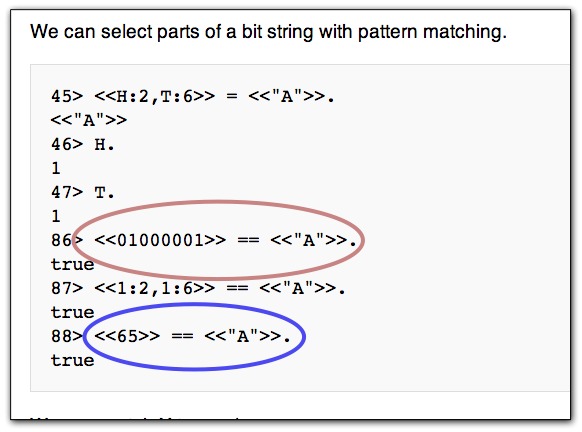You will see that in the red oval the literal bitstring syntax <<01000001>> is the same thing as the literal bitstring syntax <<”A”>>.

 The second syntax is all the rage for improving string performance in Erlang libraries.  The reason for favoring a bitstring representation <<”A”>> over regular Erlang strings “A” has to do with the the fact that regular strings are implemented as a linked-list of 32-bit integers.  Using the second syntax makes the letter “A” use 1 byte of storage versus 8-bytes. I’m not great with math, but that sounds like an 8-times decrease. If you are going to be encoding strings that are within the standard ASCII set (like the string representations of HTTP-compliant headers), which never change, then using a bitstring makes a lot more sense.

The implication of the true evaluation of the statement  is <<”A”>> == <<01000001>> is that <<01000001>> is the syntax for bits and binaries.
Look at it →   <<01000001>>.
It has 8 places and all the symbols are either 1’s or 0’s.  It must be a byte, and those 1’s and 0’s must be the bits.  Wrong.

Before, explaining why, let me explain why I thought so (and why this wiki page should be ashamed of itself for using this example), by showing those of you who have never converted a binary to a decimal what the binary number 01000001 is in decimal.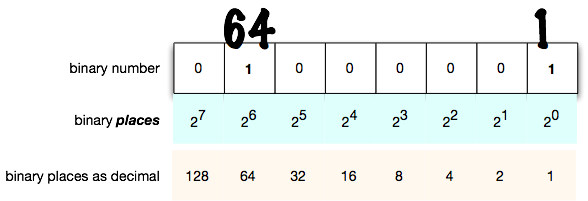In the above diagram, we have put the binary number in boxes to show how in a base-2 place-based system (or positional-notation) the 1’s are counted as that many-times the places-box.  We are so used to doing it in base-10, that we forget we are doing it.  But with base-2 you need to remember that 2 to the 6th power is the same thing as 64 (in decimal).

Seeing this algorithm for transforming a base-2 numeral to a base-10 numeral, we see obviously that 01000001 is 64 + 1 = 65.

And what is the significance of the decimal number ‘65’?

Well if you open up your ASCII table you will see that 65 is the letter “A” (it’s also 65 in UTF-8).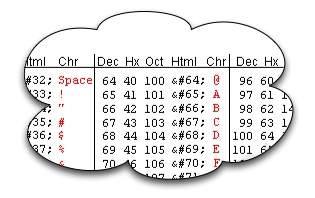Ok, well now it seems obvious that <<”A”>> == <<01000001>> evaluates to true because the <<01000001>> syntax is the same thing as 65 which is the letter “A”.  Again, WRONG.

Back to the screenshot I took on the offending site.  You will note the blue ellipse circles another true statement <<65>> == <<”A”>> . Is that contradictory?  If they are both equal to <<”A”>>, then they should be equal to each other. (Equality is, after all, transitive. I hope).

Fire up erl and you will see that they are equal to each other: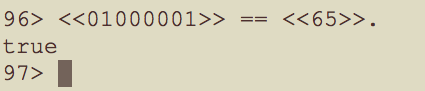So what gives?  Remember, my quest was about syntax.  So now my first reaction is to say, “ahh, the Erlang lexer must have noticed the 0’s padding within the chevrons and properly guessed that it was a syntactical binary number”.  A tiny shiver, as if a warning, passed through me, but I dismissed it and wasted 10 minutes of my life.

So, continuing down this assumption, were we to increase our binary number by 1, we should get 01000010 == 66.  Right?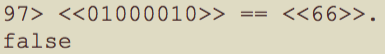Well, it says false.  So what is the problem?

Let’s use erl to evaluate  <<01000010>> directly: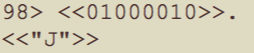Holey Smokes!  That makes no sense.  Are we saying that the letter after “A” is “J”?  Weird.

# Let’s Get to the Point

The reason that none of this makes sense, and the start of our explanation is that naked numerals within the erlang chevron syntax are treated as decimal numerals.  I purposefully used the term numerals to differentiate the symbol itself from the abstract number.  This means that the naked chevron-sandwhiched token <<01000001>> is actually a pair of chevrons surrounding the number “one million and one” and encoded as such.

## Why I made a Mistake

Earlier, I had assumed that the Erlang lexer was clever and noticed the prefixed zero padding, in order to lex <<01000001>> as a binary number.  That was my mistake.  I suppose I could make an argument that any number preceded by 0 and having only 1’s and 0’s could be interpreted as binary.  But it’s really quite a pain to implement.

## But We are Not Done

So, if the syntax of naked numerals within chevrons treats the numerals as a decimal encoding, then why does this evaluate to true?I know, for a fact, that the decimal number 65 is not equal to the decimal number 1,000,001.  We still have some weirdness to account for.

And here is the final trick, the syntax for naked numerals within chevrons is short-hand for numerals with some bit-length adornment, specifically 8.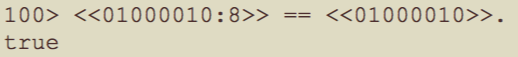You see that colon, followed by the number 8?  Well that is syntax for bitstring length.  It is stating that the decimal numeral 1,000,010 will be crammed into 8-bits.  And if the binary number is greater than 8 bits??

Ah hah!   That is the trick.  If the binary number is greater than 8-bits, then we truncate the most-significant bits and leave the 8-least-significant bits as the number.

So let’s return to the naked syntax <<01000001>>. Remember, this is shorthand for <<01000001:8>> which is saying “Convert the decimal numeral ‘one million and one’ into binary, and then truncate everything but the 8 least-significant bits.”

So what is “one million and one” in binary?
Well, the answer is 11110100001001000001

Ok, so here we go:
(0100001)base-10  is equal to  (11110100001001000001)base-2

Let’s examine the binary numeral again:
11110100001001000001
As you can see I highlighted the last 8 places (or least significant places) and we can see that our decimal numeral is found in the last eight places of the binary numeral.
(0100001)base-10  is equal to  (11110100001001000001)base-2

Again, this is why the wiki page should be ashamed of itself. It used a decimal numeral that accidentally truncates to its binary representation.  So very confusing.

# Finally

If you’re interested in not losing those more significant bits, then just change the bit-length adornment.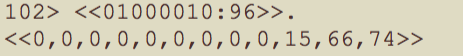Here, you see that we took our decimal numeral “one million and ten” and said “cram this into a bitstring of length 96.  You can see that we need 3-bytes worth of encoding, but the remaining 9-bytes are just zero padding.  (that 74, by the way, is the letter “J” -- and so we come full circle).

In the end,is true, but only because you are losing significant precision because of the syntax on the left.  Beware.

Enjoy.

1.Thank you for taking the time to write this post and share your findings.

2.Thanks very much, it is helpful

3.I read it today, thanks a lot, it clarified a lot for me

4.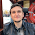Thanks for your work.

5.Thanks very much, this is very helpful me to know more about erlang.

6.Good read, At first I didn't get why it jumped to "J" from "A". I see that since it is decimal there is a difference of 9 betwee 1 and 10, hence the jump. Thanks for writing it.